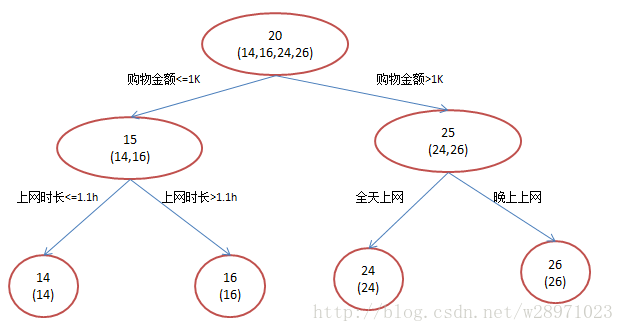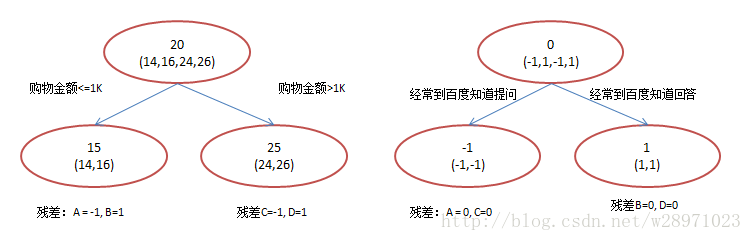@evilking 2018-05-01T10:30:23.000000Z 字数 12383 阅读 2475

机器学习篇

# GBDT（梯度提升决策树）

## DT（决策回归树）

我们知道 分类树在每次分枝时，是穷举每一个 feature 的每一个阈值，找到使得按照 feature <= 阈值和feature > 阈值分成两枝的熵最大的 feature 和阈值，按照该标准分枝得到的两个新节点，按同样的方法递归分裂下去，直到所有样本都被分入唯一的叶子节点，或达到预设的终止条件（如果叶子节点的样本不唯一，则以多数类作为叶子节点的分类结果）.


不过在每个节点（不一定是叶子节点）都会得到一个预测值，以人的年龄为例，该预测值等于属于这个节点的所有人的年龄的平均值。分枝时穷举每一个feature的每个阈值找最好的分割点，但衡量最好的标准不再是最大熵，而是最小化均方差--即（每个人的年龄-预测年龄）平方的总和除以 N（总人数），或者说是每个人的预测误差平方和 除以 N。这很好理解，被预测出错的人数越多，错的越离谱，均方差就越大，通过最小化均方差能够找到最靠谱的分枝依据。直到每个叶子节点上人的年龄都唯一，或者达到预设终止条件，若最终叶子节点上的人的年龄不唯一，就以该节点上所有人的平均年龄作为该叶子节点的预测值.


## GBDT 实例

GBDT的核心就在于，每一棵树学的是之前所有树结论和的残差，这个残差就是一个加预测值后能得真实值的累加量A: 14 岁高一学生，购物较少，经常问学长问题；预测年龄 $A = 15 + (– 1) = 14$

B: 16 岁高三学生，购物较少，经常被学弟问问题；预测年龄 $B = 15 + 1 = 16$

C: 24 岁应届毕业生，购物较多，经常问师兄问题；预测年龄 $C = 25 + (–1) = 24$

D: 26 岁工作两年员工，购物较多，经常被师弟问问题；预测年龄 $D = 25 + 1 = 26$

## GBDT 深入理解

### Boosting

1. 给定一个起始点 $x_0$
2. $i = 1,2,\cdots,K$ 分别做如下迭代:
$\qquad x_i = x_{i-1} + \gamma_{i-1} \times g_{i-1}$
其中 $g_{i-1} = -\frac{\partial f}{\partial x} |_{x = x_{i-1}}$ 表示 $f$$x_{i-1}$ 点的梯度

3. 直到 $|g_{i-1}|$ 足够小，或者是 $|x_i - x_{i-1}|$ 足够小

1. 选择一个起始常量函数 $f_0$
2. $i=1,2,\cdots,K$ 分别做如下迭代:

(a) 计算离散梯度值 $\hat{g}_{i-1}(x_j) = -\frac{\partial L}{\partial F(x_j)}|_{F = F_{i-1}},j = 1,2,\cdots,N$

(b) 对 $\hat{g}_{i-1}(x_j)$ 做函数拟合得到 $g_{i-1}$

(c) 通过 line search 得到 $\gamma_{i-1} = arg \ \min L(F_{i-1} + \gamma_{i-1} g_{i-1})$

(d) 令 $F_i = F_{i-1} + \gamma_{i-1} g_{i-1}$

3. 直到 $|\hat{g}_{i-1}|$ 足够小，或者迭代次数完毕

## GBDT 的推导

GBDT 原始版本的算法框架:

1. 初始化 $f_0$
2. $for \quad k = 1 \ to \ K \quad do$
3. $\qquad \hat{y}_i = -\frac{\partial L(y_i,F_{k-1}(x_i))}{\partial F_{k-1}},i = 1,2,\cdots,N$
4. $\qquad \{R_j,b_j\}_1^{J^*} = arg \min_{\{R_j,b_j\}_1^J} \sum_{i=1}^N \left[ \hat{y}_i - f_k(x_i;\{R_j,b_j\}_1^J) \right]^2$
5. $\qquad \begin{array} & \rho^{*} &= arg \min_{\rho} L\left( \{ y_i,F_{k-1}(x_i) + \rho f_k(x_i) \}_1^N \\ = arg \min_{\rho} \sum_{i=1}^N L\left( y_i,F_{k-1}(x_i) + \rho f_k(x_i) \right) + \Omega(f_k) \right) \end{array}$
6. $\qquad 令 \ f_k = \rho^* f_k,F_k = F_{k-1} + f_k$
7. $end$

• $\{(x_i,y_i)\}_1^N$ 表示 $N$ 个样本；

• $f_k$ 表示第 $k$ 棵决策树，这棵决策树的预测值为 $f_k = \sum_{j=1}^J b_j$

• $K$ 表示总共要生成的决策树数量；

• $J$ 表示叶子节点的数量；

• $F_{k-1}(x_i)$ 表示前 $k-1$ 棵决策树综合得到的结果，即 $F_{k-1} = \sum_{i=1}^{k-1} f_i$

• $L(y_i,F_{k-1}(x_i))$ 表示损失函数；

• $\{R_j,b_j\}_1^J$ 表示一个划分，$R_j$ 表示叶子节点 $j$ 上的样本集合，$b_j$ 表示该叶子节点上的样本输出值.

GBDT 就是一个不断拟合响应（比如残差）并叠加到 $F$ 上的过程，在这个过程中，残差不断减小，$Loss$ 不断接近最小值.

• 问题一: 如果已经得到了 $\{R_j\}_1^J$，最小化 $\cal{L}_k$$\{b_j\}_1^J$ 是多少？
这个问题是为了在已经得到叶子节点的情况下，得到的最优目标函数值是多少.

• 问题二: 如果将当前节点 $R_i$ 分裂，应该在哪一个分裂点使得 $\cal{L}_k$ 最小？
这个问题是为了解如何进行节点分裂.

• 问题一
这个比较好求，直接目标函数 $\cal{L}_k(\{b_j\}_1^J,\{R_j\}_1^J)$$b_j$ 求导，令结果为零:

所以得:
此时最小的 $\cal{L}_k$ 是:

• 问题二
$\{R_j\}_1^J$ 的方法是对输入 $x$ 所属空间的一种划分方法，不连续，无法求导. 取而代之的是使用贪心算法，即分裂某节点时，只考虑对当前节点分裂后，哪个分裂方案能得到最小的 $\cal{L}_k$ .

假设欲将当前节点 $R_j$ 分裂成 $R_L,R_R$ 这两个子节点，且要使得分裂后整棵树的 $\cal{L}_k$ 最小；而整棵树的最小 $\cal{L}_k$ 等于每个叶子节点上最小的损失的和；又由于对节点 $R_j$ 的分裂过程只涉及到三个节点———— $R_j,R_L,R_R$，所以这个目标可以换一种描述.

这个问题的目标可以描述为: